# Include Mathematical Memes Into Your Routine!

### Include Mathematical Memes Into Your Routine!

K. Elizabeth Hammonds Auburn University hammoke@auburn.edu

W. Gary Martin Auburn University martiwg@auburn.edu

A meme is a digital form of communication found on social media, typically in the form of a digital cartoon or visual image that makes a humorous point (Knobel & Lankshear, 2007). Mathematical memes can communicate ideas, beliefs, or attitudes about mathematics or mathematics concepts (Bini & Rubutti, 2019, Bini et al., 2020; Hall, 1997), as shown in Figure 1. Internet memes are created by users according to collective established and shared rules (Osterroth, 2018), and these images have the potential to positively or negatively impact one’s beliefs about the nature of mathematics (Barba, 2018; Wilson & Laterrell, 2001). Furthermore, mathematical memes provide opportunities for students to construct mathematical knowledge and communicate digitally (Bini et al., 2020).

Figure 1

Examples of Mathematical Memes Found on the Internet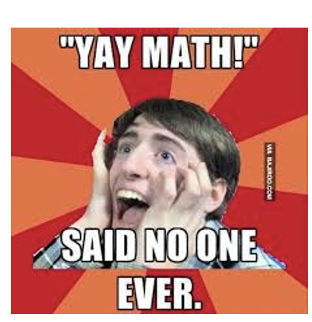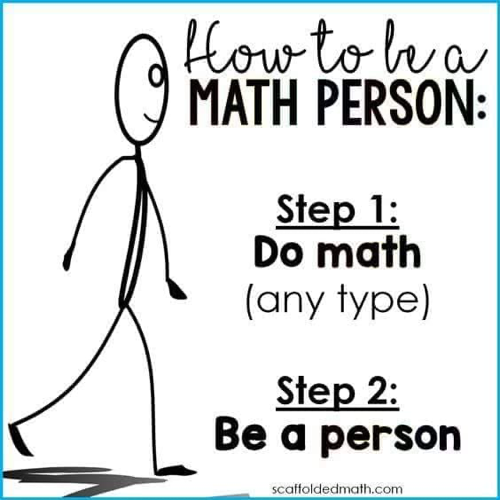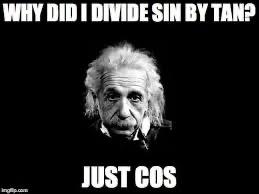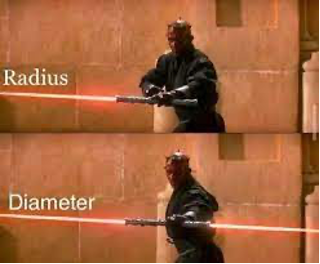The representation of mathematical content in memes is different from formal mathematical representations; mathematical memes are mathematical statements, but their decoding requires additional knowledge from the memesphere (Bini et al., 2020). Mathematical memes have three levels of conveying meaning: structural, social, and specialized (Bini & Rubutti, 2019). More information on meme levels is contained in Table 1.

Table 1

Meme Levels (Bini & Rubutti, 2019)

 Level Definition Structural Meme structure and graphics. Social The shared culture connected to the viral images and grammar. Specialized The mathematical symbols and text.

Only a deep understanding of the encoded mathematics reveals the power of the meme, and analyzing the meme’s decoding process must be situated and understood through the interactions among its members (Bini & Rubutti, 2019). For example, the meme message in Figure 2 can be decoded only if the viewer knows the binomial expansion and is knowledgeable of the common misconception (Bini & Rubutti, 2019).

Figure 2

Mathematical Content Meme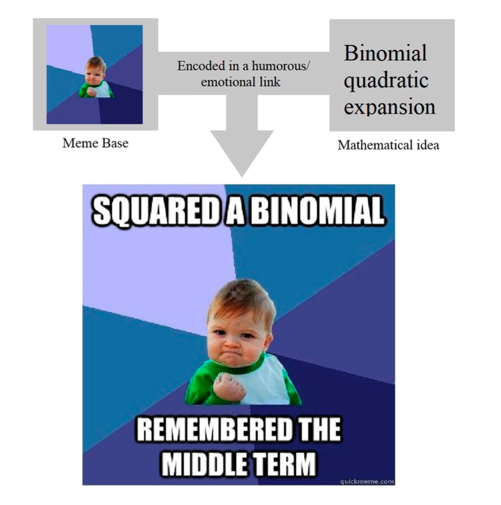Memes promote classroom discourse around both mathematical content and mathematical beliefs (Bin & Rubutti, 2019, Penland, 2021), because mathematical memes contain images connecting to human emotions concerning mathematics. Furthermore, memes have been shown to influence students’ mathematical identities (Penland, 2021; Bini et al., 2020). For example, Penland (2021) allowed students to post a meme as a valid way to participate in the online class discussion and earn participation credit. For the first half of the semester students mainly posted negative memes about mathematics – mathematics as useless, confusing, or even punitive (Penland, 2021). Toward the end of the semester, students submitted fewer negative mathematical memes. The memes produced a student-directed entry point to open discussions about unproductive views of mathematics as well as injected emotions, often humor, to deflect the intimidating nature of mathematics. The use of memes in mathematics classrooms has the potential to help reduce disinterest in the subject and increase student motivation.

Bini and Rubutti (2019) used memes to cultivate classroom discourse around mathematics content with high school students. Students created memes on one of the course’s mathematics standards and posted the memes in a collective online space. This allowed the researchers and classroom teacher to harness the digital world to gain an understanding into student’s mathematical identity; the student-created memes highlighted the topics students’ consciously selected and felt comfortable with as well as the ways in which the students tried to explain the meme and concept clearly (Bini et al., 2020). According to Bini and Rubutti (2019), allowing students to leverage mathematical memes and popular culture to engage in mathematical practice and justification can build a more open inclusive classroom by honoring diverse ways of contributing to the mathematics classroom, which aligns with AMTE’s (2017) recommendation that well-prepared beginning mathematics teachers must consider both the mathematics content and the context of a task in order to position learners as doers of mathematics.

Implications for Mathematics Teacher Educators

As mathematics teacher educators (MTE), we need to prepare teachers who “recognize the fast rate at which technologies emerge and are committed to staying abreast of new tools” (NCTM, 2012, p. 3) and embrace these new ways of communicating through technology, including mathematical memes found on the Internet. MTEs can explore how to incorporate these mathematical memes as part of their classroom routines, since well-prepared teachers of mathematics need to be proficient with technology designed to support mathematical reasoning and sense making (AMTE, 2017). Implementing memes into the classroom opens avenues for MTEs to model for prospective teachers “a classroom community in which students present ideas, challenge one another, and construct meaning together; varied mathematical strengths are valued and celebrated” (AMTE, 2017, p. 13). As we use memes to engage our prospective teachers in discussions around mathematical beliefs and academic content, we can help them see how they can help their future students to harness their creative strengths to express themselves in digital media appropriate to their goals (Serano, 2016) as well as model AMTE’s goal to foster “the mathematical dispositions of students to include confidence, flexibility, perseverance, curiosity, self-monitoring, and appreciation of mathematics'' (AMTE, 2017, p. 20).

In addition, mathematical memes can drive mathematical discussions to help foster an understanding of and interest in mathematics (Bini & Rubutti, 2019; Penland, 2021). Adding memes to classroom routines allows MTEs to model taking “conscious and intentional actions to build students’ agency as mathematical learners” (AMTE, 2017, p. 13). The sometimes humorous nature of mathematical memes can potentially create supportive environments by minimizing the daunting nature of mathematics and thus, allowing MTEs and their students to engage in discourse around mathematics and mathematical beliefs. Implementing memes into the classroom to intentionally discuss mathematical beliefs can potentially address the AMTE (2017) Standard highlighting ethical practice and advocacy; well-prepared beginner teachers “recognize the need to challenge…the messages conveyed to students and the greater public through school mathematics” (p. 24). In conclusion, we suggest that mathematics teacher educators consider extending the types of work done with students in the PK-12 setting using mathematical memes (Bini & Rubutti, 2019; Bini et al., 2020) to preservice teacher education, both in helping prospective teachers of mathematics build their mathematical identities and in modeling a potentially useful tool that they could use in their mathematics instruction.

References

Association of Mathematics Teacher Educators (AMTE). (2017). Standards for preparing teachers of mathematics. AMTE.

Barba, K. (2020). The mathematical mindsets and mathematical identities revealed in social media discourse. Journal of Mathematics Education at Teachers College, 11(2), 23-34.

Bini, G., & Rubutti, O. (2019, February). Meanings in mathematics: Using internet memes and augmented reality to promote mathematical discourse [Conference paper]. Eleventh Congress of the European Society for Research in Mathematics Education, Utrecht, Netherlands.

Bini, G., Robutti, O., & Bikner-Ahsbahs, A. (2020). Maths in the time of social media: Conceptualizing the internet phenomenon of mathematical memes. International Journal of Mathematical Education in Science and Technology: 1-39.

Hall, S. (Ed.). (1997). Representation: Cultural representations and signifying practices. Sage.

Knobel, Michele & Lankshear, Colin. (2007). Online memes, affinities, and cultural production. In M. Knobel & C. Lankshear (Eds.), A new literacies sampler (pp. 199–227). Peter Lang Publishing.

National Council of Teachers of Mathematics. (2012). NCTM CAEP mathematics content for secondary: Addendum to the NCTM CAEP Standards 2012. NCTM.

Osterroth, A. (2018). Semiotics of internet memes. ResearchGate. https://doi.org/10.13140/RG.2.2.12320.89605.

Penland, A. (2022). Mathematical memes: Fun and insightful. MMA: Math Values. https://www.mathvalues.org/masterblog/mathematical-memes-fun-and-insightful

Serano, S. (2021). 5 ways to use memes with students. International Society for Technology in Education (ISTE). https://www.iste.org/explore/classroom/5_ways_use_memes_students

Wilson, J. L., & Laterrell, C. M. (2001). Nerds or nuts? Pop culture portrayals of mathematicians. ETC: A Review of General Semantics, 58(2). 172-178.